Tim's Blog

## Finding Approximations For Functions Numerically

09 Jun 2018

It’s hard to compute some functions, like trig and special functions, hence why approximations are frequently used. Sometimes, it may be the only way to calculate these functions. Mathematicians have worked on these approximations for centuries, including many famous ones such as the small angle approximation and Taylor series to many high precision methods such as Chebyshev polynomials. However, when using approximations for problems done by hand you want to both use a simple but accurate approximation, which usually means Taylor series or something similar. The problem with this is that some Taylor series require many higher order terms to converge to the desired accuracy. What if approximations in the form of a second degree polynomial divided by another second degree polynomial existed for many functions?

It’s hard to compute some functions, like trig and special functions, hence why approximations are frequently used. Sometimes, it may be the only way to calculate these functions. Mathematicians have worked on these approximations for centuries, including many famous ones such as the small angle approximation and Taylor series to many high precision methods such as Chebyshev polynomials. However, when using approximations for problems done by hand you want to both use a simple but accurate approximation, which usually means Taylor series or something similar. The problem with this is that some Taylor series require many higher order terms to converge to the desired accuracy. What if approximations in the form of a second degree polynomial divided by another second degree polynomial existed for many functions?

An approximation like that could be expressed as,

where $a$, $b$, $c$, $d$, $e$, and $f$ are all constants. An approximation of this form would be ideal for doing calculations by hand because polynomials are easy to remember and work with and having two-second degree polynomials divided by one another could capture the more complex curves in some functions. The general strategy for finding these approximations will be to use scipy’s curve_fit function to fit the expression above to the desired function and then do some light manipulating by hand to get pretty and easy to remember integer coefficients if possible.

import numpy as np
import scipy.constants as c
from scipy.optimize import curve_fit
import matplotlib.pyplot as plt

ysize = 7.0
xsize = 7.0


### Sine

Let’s start with $\sin\left(\theta\right)$. It would be nice if an approximation could be found that would work on the interval $\left(-\frac{\pi}{2},\frac{\pi}{2}\right)$. To give an idea for the current approximations that are commonly used I’ve made a graph of $\sin\left(\theta\right)$ and the desired interval with its first, second, and third order Taylor series.

theta = np.linspace(-c.pi/2.0, c.pi/2.0, 100)
sin_theta = np.sin(theta)
taylor1 = theta
taylor2 = taylor1 - np.power(theta, 3.0)/6.0
taylor3 = taylor2 + np.power(theta, 5.0)/120.0

fig, ax = plt.subplots(1, 1)
fig.set_size_inches(xsize, ysize)
ax.plot(theta, sin_theta, label = r"$\sin\left(\theta\right)$")
ax.plot(theta, taylor1, label = r"$\theta$")
ax.plot(theta, taylor2, label = r"$\theta-\frac{\theta^3}{3!}$")
ax.plot(theta, taylor3, label = r"$\theta-\frac{\theta^3}{3!}+\frac{\theta^5}{5!}$")
ax.axhline(y = 0.0, color = "k", linestyle = ":")
ax.set_title("Comparision of Approximations for "+r"$\sin\left(\theta\right)$")
ax.set_xlabel(r"$\theta$"+" in Radians")
ax.legend()
plt.show()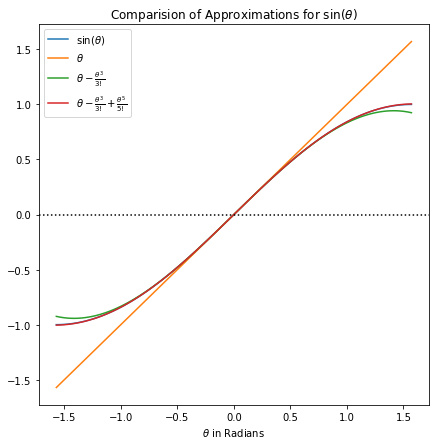The graph above shows that the small angle approximation $\sin\left(\theta\right)\approx\theta$ is good for about $\pm\frac{1}{4}$radians, but the second degree Taylor series is a good approximation for $\pm1$radians. However, to capture $\sin\left(\theta\right)$ on the interval $\left(-\frac{\pi}{2},\frac{\pi}{2}\right)$ a third-degree Taylor series is required. Now to see if an approximation in the form of second-degree polynomial divided by a second-degree polynomial would be any use here.

def sin_approximation(theta, a, b, c, d, e, f):
return (a * np.power(theta, 2.0) + b * theta + c)/(d * np.power(theta, 2.0) + e * theta + f)

popt, pcov = curve_fit(sin_approximation, theta, sin_theta)
print(popt)

[ 1.82584309e-05  4.20858544e+00 -3.37675226e-08  9.67256862e-01
2.20126431e-05  4.08214643e+00]


Those coefficients are very messy, and the whole point of this exercise was to develop easy to remember high accuracy approximations for doing pen and paper calculations with. To simplify that expression let the small coefficients go to zero, multiply top and bottom by $5$, and then round. This yields the following, actually quite nice looking, approximation.

fig, (ax1, ax2) = plt.subplots(1, 2)
fig.set_size_inches(2.0 * xsize, ysize)

ax1.plot(theta, sin_theta, label = r"$\sin\left(\theta\right)$")
ax1.plot(theta, sin_approximation(theta, *popt), label = "Numerical Approximation")
ax1.plot(theta, sin_approximation(theta, 0.0, 21.0, 0.0, 5.0, 0.0, 20.0), label = "Nice Approximation")
ax1.set_title("Comparision of New Approximation for "+r"$\sin\left(\theta\right)$")
ax1.set_xlabel(r"$\theta$"+" in Radians")
ax1.legend()

ax2.plot(theta, np.absolute(sin_theta - sin_approximation(theta, *popt)), label = "Numerical Approximation")
ax2.plot(theta, np.absolute(sin_theta - sin_approximation(theta, 0.0, 21.0, 0.0, 5.0, 0.0, 20.0)), label = "Nice Approximation")
ax2.set_title("Approximation Error")
ax2.set_xlabel(r"$\theta$"+" in Radians")
ax2.legend()

plt.show()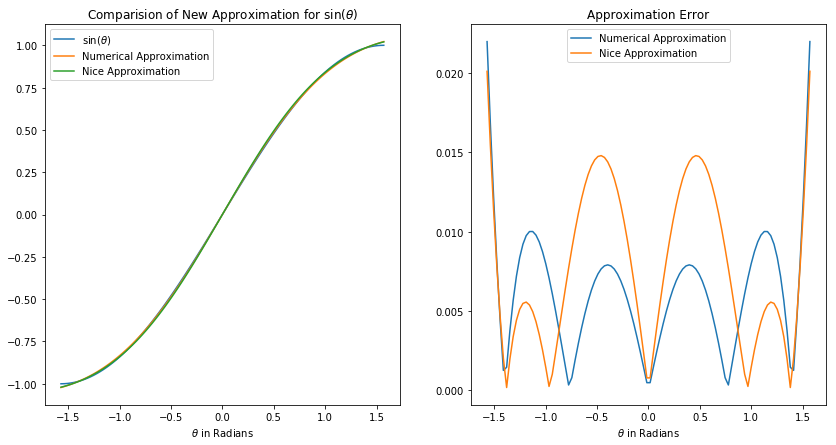On the graph on the left above there is a plot of the original approximation found from the curve fit as well as a plot of the cleaned up “nice” version of the approximation. The graph on the right above shows the error in both the original approximation and the nice approximation. This error approximation graph really shows that the nice approximation actually does a great job at approximation $\sin\left(\theta\right)$ on the desired interval. At the fringes of the interval, both the original and nice approximation do have larger errors, unfortunately, so it is not quite as good as the third-degree Taylor series. However, the cost paid in accuracy is more than made up for in the approximation’s simplicity.

### Cosine

Now to work on $\cos\left(\theta\right)$, using the same interval and methodology as before.

theta = np.linspace(-c.pi/2.0, c.pi/2.0, 100)
cos_theta = np.cos(theta)
taylor1 = np.ones(100)
taylor2 = taylor1 - np.power(theta, 2.0)/2.0
taylor3 = taylor2 + np.power(theta, 4.0)/24.0

fig, ax = plt.subplots(1, 1)
fig.set_size_inches(xsize, ysize)
ax.plot(theta, cos_theta, label = r"$\cos\left(\theta\right)$")
ax.plot(theta, taylor1, label = r"$1$")
ax.plot(theta, taylor2, label = r"$1-\frac{\theta^2}{2!}$")
ax.plot(theta, taylor3, label = r"$1-\frac{\theta^2}{2!}+\frac{\theta^4}{4!}$")
ax.axhline(y = 0.0, color = "k", linestyle = ":")
ax.set_title("Comparision of Approximations for "+r"$\cos\left(\theta\right)$")
ax.set_xlabel(r"$\theta$"+" in Radians")
ax.legend()
plt.show()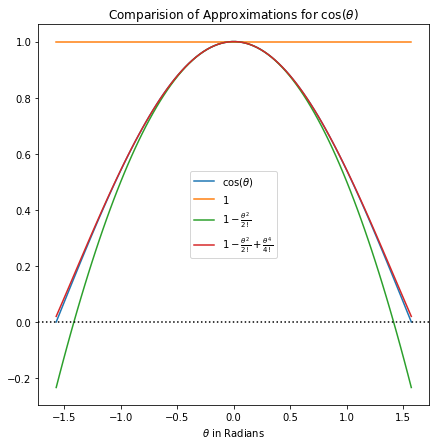$\cos\left(\theta\right)$ will be a unique challenge as can be seen from the graph above because not even a third-degree polynomial can fully capture its behavior. However, that only gives all the more reason to develop a new approximation for this interval.

def cos_approximation(theta, a, b, c, d, e, f):
return (a * np.power(theta, 2.0) + b * theta + c)/(d * np.power(theta, 2.0) + e * theta + f)

popt, pcov = curve_fit(cos_approximation, theta, cos_theta)
print(popt)

[-6.67768965e-01  4.66558341e-07  1.64387171e+00  1.64007775e-01
1.98276010e-06  1.64275682e+00]


Those numbers are a similar situation to those for the $\sin\left(\theta\right)$ approximation. I will use the same procedure as before to simplify the fraction but instead multiply top and bottom by $6$ instead of $5$. This yields an another very nice and easy to remember approximation.

fig, (ax1, ax2) = plt.subplots(1, 2)
fig.set_size_inches(2.0 * xsize, ysize)

ax1.plot(theta, cos_theta, label = r"$\sin\left(\theta\right)$")
ax1.plot(theta, cos_approximation(theta, *popt), label = "Numerical Approximation")
ax1.plot(theta, -cos_approximation(theta, 2.0, 0.0, -10.0, 1.0, 0.0, 10.0), label = "Nice Approximation")
ax1.set_title("Comparision of New Approximation for "+r"$\cos\left(\theta\right)$")
ax1.set_xlabel(r"$\theta$"+" in Radians")
ax1.legend()

ax2.plot(theta, np.absolute(cos_theta - cos_approximation(theta, *popt)), label = "Numerical Approximation")
ax2.plot(theta, np.absolute(cos_theta + cos_approximation(theta, 4.0, 0.0, -20.0, 2.0, 0.0, 20.0)), label = "Nice Approximation")
ax2.set_title("Approximation Error")
ax2.set_xlabel(r"$\theta$"+" in Radians")
ax2.legend()

plt.show()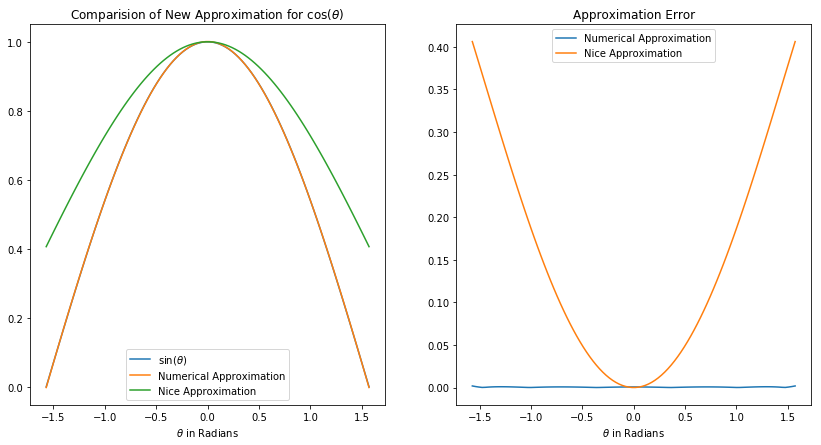It seems that multiplying top and bottom by $6$ and rounding is not sufficient, because that’s a pretty bad approximation. Yet, the original approximation from the curve fit works quite well, which indicates that an approximation of the desired form will still fit well. I just have to be careful about rounding since this fit seems to be particularly sensitive to even slight changes. Trying the procedure above again with $31$ instead of $6$ yields the following approximation, which is a little bit more complicated than before.

fig, (ax1, ax2) = plt.subplots(1, 2)
fig.set_size_inches(2.0 * xsize, ysize)

ax1.plot(theta, cos_theta, label = r"$\cos\left(\theta\right)$")
ax1.plot(theta, cos_approximation(theta, *popt), label = "Numerical Approximation")
ax1.plot(theta, -cos_approximation(theta, 24.0, 0.0, -59.0, 6.0, 0.0, 59.0), label = "Nice Approximation")
ax1.set_title("Comparision of New Approximation for "+r"$\cos\left(\theta\right)$")
ax1.set_xlabel(r"$\theta$"+" in Radians")
ax1.legend()

ax2.plot(theta, np.absolute(cos_theta - cos_approximation(theta, *popt)), label = "Numerical Approximation")
ax2.plot(theta, np.absolute(cos_theta + cos_approximation(theta, 24.0, 0.0, -59.0, 6.0, 0.0, 59.0)), label = "Nice Approximation")
ax2.set_title("Approximation Error")
ax2.set_xlabel(r"$\theta$"+" in Radians")
ax2.legend()

plt.show()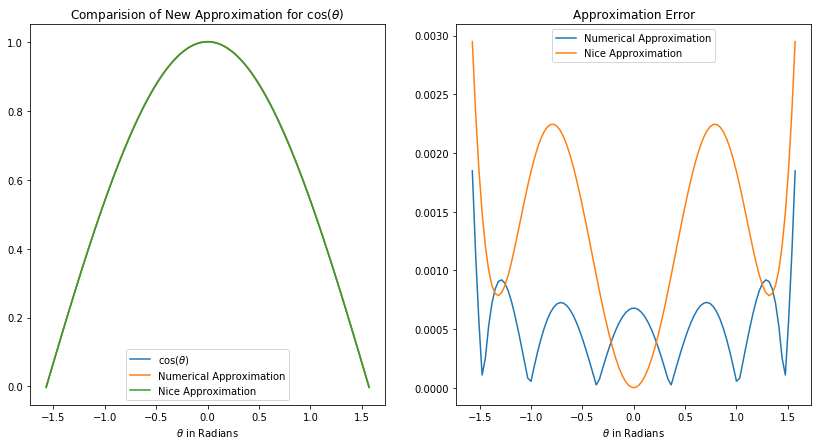Yet, it appears that the extra complexity paid off because the error in this approximation for $\cos\left(\theta\right)$ is a whole order of magnitude smaller than the error in the approximation for $\sin\left(\theta\right)$ above. This will make this approximation perfect for doing high precision problems by hand.

### Tangent

Now to work on $\tan\left(\theta\right)$. This time on the interval $\left(-\frac{2\pi}{5},\frac{2\pi}{5}\right)$, since tangent diverges at $\frac{\pi}{2}$ and $-\frac{\pi}{2}$ and attempting to capture asymptotic behavior with an approximation is extremely difficult.

theta = np.linspace(-2.0*c.pi/5.0, 2.0*c.pi/5.0, 100)
tan_theta = np.tan(theta)
taylor1 = theta
taylor2 = taylor1 + np.power(theta, 3.0)/3.0
taylor3 = taylor2 + 2.0*np.power(theta, 5.0)/15.0

fig, ax = plt.subplots(1, 1)
fig.set_size_inches(xsize, ysize)
ax.plot(theta, tan_theta, label = r"$\tan\left(\theta\right)$")
ax.plot(theta, taylor1, label = r"$\theta$")
ax.plot(theta, taylor2, label = r"$\theta-\frac{\theta^3}{3}$")
ax.plot(theta, taylor3, label = r"$\theta-\frac{\theta^3}{3}+\frac{2\theta^5}{15}$")
ax.axhline(y = 0.0, color = "k", linestyle = ":")
ax.set_title("Comparision of Approximations for "+r"$\tan\left(\theta\right)$")
ax.set_xlabel(r"$\theta$"+" in Radians")
ax.legend()
plt.show()This graph demonstrates a little bit of the asymptotic behavior being difficult to capture like I mentioned before. Even a third-degree Taylor series (which in this case is a fifth-degree polynomial!) can’t even capture this behavior and is only accurate for $\pm\frac{4}{5}$radians. Perhaps the approximation in the form that I’m searching for will be able to handle that behavior on this interval.

def tan_approximation(theta, a, b, c, d, e, f):
return (a * np.power(theta, 2.0) + b * theta + c)/(d * np.power(theta, 2.0) + e * theta + f)

popt, pcov = curve_fit(tan_approximation, theta, tan_theta)
print(popt)

[-3.91210361e-06  6.23897599e-01  1.37220488e-06 -2.44024306e-01
-1.21901808e-06  6.42206359e-01]


Unfortunately, there is no great multiplier for this set of coefficients, but the smallest one that does come the closest to yield integer coefficients is $5$. This yields the approximation below.

fig, (ax1, ax2) = plt.subplots(1, 2)
fig.set_size_inches(2.0 * xsize, ysize)

ax1.plot(theta, tan_theta, label = r"$\tan\left(\theta\right)$")
ax1.plot(theta, tan_approximation(theta, *popt), label = "Numerical Approximation")
ax1.plot(theta, -tan_approximation(theta, 0.0, 3.0, 0.0, 1.0, 0.0, -3.0), label = "Nice Approximation")
ax1.set_title("Comparision of New Approximation for "+r"$\tan\left(\theta\right)$")
ax1.set_xlabel(r"$\theta$"+" in Radians")
ax1.legend()

ax2.plot(theta, np.absolute(tan_theta - tan_approximation(theta, *popt)), label = "Numerical Approximation")
ax2.plot(theta, np.absolute(tan_theta + tan_approximation(theta, 0.0, 3.0, 0.0, 1.0, 0.0, -3.0)), label = "Nice Approximation")
ax2.set_title("Approximation Error")
ax2.set_xlabel(r"$\theta$"+" in Radians")
ax2.legend()

plt.show()The nice approximation did much better than I was originally expecting given the concern I had about finding nice coefficients, which is a nice surprise. However, the nice approximation, like most approximations for $\tan\left(\theta\right)$, is not great at capturing the asymptotic behavior which makes it a less than ideal approximation for the interval $\left(-\frac{2\pi}{5},\frac{2\pi}{5}\right)$. On the interval, $\left(-\frac{3\pi}{4},\frac{3\pi}{4}\right)$ this approximation is very good, so I’ll take it, especially since it beats using a third-degree Taylor series.

### Arcsine

Now for $\sin^{-1}\left(x\right)$, which is a more specialized function with “asymptotic-like” behavior near $x=\pm1$. For this approximation I’ll try to find something on the interval $\left(-1, 1\right)$, which is the entire domain of $\sin^{-1}\left(x\right)$.

x = np.linspace(-1.0, 1.0, 100)
arcsin_x = np.arcsin(x)
taylor1 = x
taylor2 = taylor1 + np.power(x, 3.0)/6.0
taylor3 = taylor2 + 3.0*np.power(x, 5.0)/40.0

fig, ax = plt.subplots(1, 1)
fig.set_size_inches(xsize, ysize)
ax.plot(x, arcsin_x, label = r"$\sin^{-1}\left(x\right)$")
ax.plot(x, taylor1, label = r"$x$")
ax.plot(x, taylor2, label = r"$x-\frac{x^3}{6}$")
ax.plot(x, taylor3, label = r"$x-\frac{x^3}{6}+\frac{3x^5}{40}$")
ax.axhline(y = 0.0, color = "k", linestyle = ":")
ax.set_title("Comparision of Approximations for "+r"$\sin^{-1}\left(x\right)$")
ax.set_xlabel(r"$x$")
ax.legend()
plt.show()This graph illustrates the “asymptotic-like” behavior that I was talking about above. Furthermore, it is interesting to note that for $\sin^{-1}\left(x\right)$ it appears that the Taylor series is slow to converge to the actual behavior. This means that if there is an approximation of the form that I’m looking for it would be many times more useful than a Taylor series approximation.

def arcsin_approximation(x, a, b, c, d, e, f):
return (a * np.power(x, 2.0) + b * x + c)/(d * np.power(x, 2.0) + e * x + f)

popt, pcov = curve_fit(arcsin_approximation, x, arcsin_x)
print(popt)

[ 4.19420562e-02  6.72733583e+02  3.38142426e-05 -2.50862024e+02
3.29547484e-02  7.25762631e+02]


The curve fit for this function produced numbers that are quite large. Instead of finding a suitable number to multiply the top and bottom by I’ll find a number to divide the top and bottom by. Luckily it seems that coefficients that matter are close to a multiple of $25$ so I’ll just divide by that. Which yields the approximation below.

fig, (ax1, ax2) = plt.subplots(1, 2)
fig.set_size_inches(2.0 * xsize, ysize)

ax1.plot(x, arcsin_x, label = r"$\sin^{-1}\left(x\right)$")
ax1.plot(x, arcsin_approximation(x, *popt), label = "Numerical Approximation")
ax1.plot(x, arcsin_approximation(x, 0.0, 27.0, 0.0, -10.0, 0.0, 29.0), label = "Nice Approximation")
ax1.set_title("Comparision of New Approximation for "+r"$\sin^{-1}\left(x\right)$")
ax1.set_xlabel(r"$x$")
ax1.legend()

ax2.plot(x, np.absolute(arcsin_x - arcsin_approximation(x, *popt)), label = "Numerical Approximation")
ax2.plot(x, np.absolute(arcsin_x - arcsin_approximation(x, 0.0, 27.0, 0.0, -10.0, 0.0, 29.0)), label = "Nice Approximation")
ax2.set_title("Approximation Error")
ax2.set_xlabel(r"$x$")
ax2.legend()

plt.show()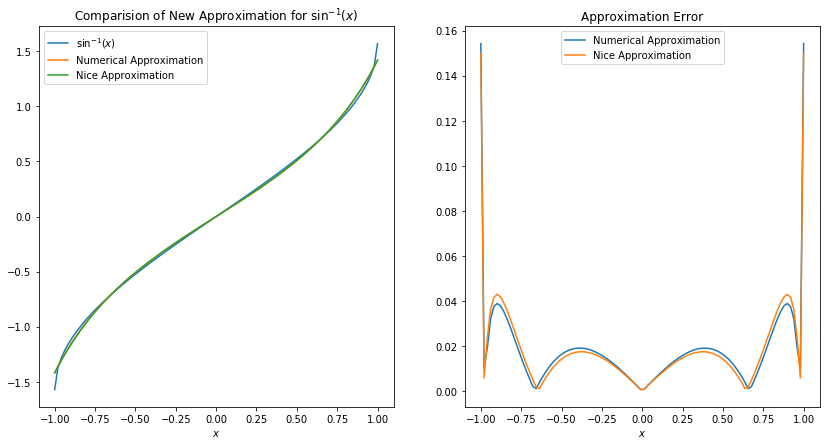Since the coefficients found by the curve fit were quite large originally, rounding and then simplifying only has a small impact on the error of the nice approximation compared to the original approximation. In fact, it seems that the nice approximation is better than the original approximation on the interval $\left(-\frac{3}{5},\frac{3}{5}\right)$ in terms of error. Both the original and nice approximation both suffer from not being able to capture the “asymptotic-like” very well but are much better at it then the Taylor series approximation.

### Logarithm

For $\ln\left(1+x\right)$ I’ll look for a suitable approximation on the interval $\left(-0.99, 1\right)$ since the most common use of an approximation for $\ln\left(1+x\right)$ is around $x=0$.

x = np.linspace(-0.99, 1.0, 100)
ln_x = np.log(1 + x)
taylor1 = x
taylor2 = taylor1 - np.power(x, 2.0)/2.0
taylor3 = taylor2 + np.power(x, 3.0)/3.0

fig, ax = plt.subplots(1, 1)
fig.set_size_inches(xsize, ysize)
ax.plot(x, ln_x, label = r"$\ln\left(1+x\right)$")
ax.plot(x, taylor1, label = r"$x$")
ax.plot(x, taylor2, label = r"$x-\frac{x^2}{2}$")
ax.plot(x, taylor3, label = r"$x-\frac{x^2}{2}+\frac{x^3}{3}$")
ax.axhline(y = 0.0, color = "k", linestyle = ":")
ax.set_title("Comparision of Approximations for "+r"$\ln\left(1+x\right)$")
ax.set_xlabel(r"$x$")
ax.legend()
plt.show()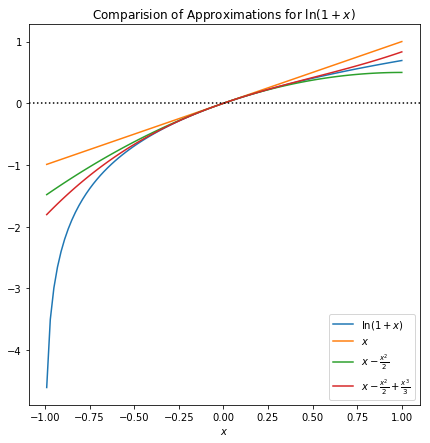Once again, it appears that $\ln\left(1+x\right)$ is a function with a Taylor series that does not like to converge, which will probably make finding a suitable approximation difficult.

def ln_approximation(x, a, b, c, d, e, f):
return (a * np.power(x, 2.0) + b * x + c)/(d * np.power(x, 2.0) + e * x + f)

popt, pcov = curve_fit(ln_approximation, x, ln_x)
print(popt)

[-897.40968348   44.90906117   11.11860947 -800.22479606 -889.46130122
142.40663397]


This is the first approximation so far that has no near-zero coefficients. Furthermore, the coefficients are all quite large, to simplify I’ll round each one to the nearest multiple of $5$ and divide through by $5$. Unfortunately, this approximation doesn’t seem like that easy of one to remember this time.

fig, (ax1, ax2) = plt.subplots(1, 2)
fig.set_size_inches(2.0 * xsize, ysize)

ax1.plot(x, ln_x, label = r"$\ln\left(1+x\right)$")
ax1.plot(x, ln_approximation(x, *popt), label = "Numerical Approximation")
ax1.plot(x, ln_approximation(x, 180.0, -9.0, -2.0, 160.0, 178.0, -28.0), label = "Nice Approximation")
ax1.set_title("Comparision of New Approximation for "+r"$\ln\left(1+x\right)$")
ax1.set_xlabel(r"$x$")
ax1.legend()

ax2.plot(x, np.absolute(arcsin_x - ln_approximation(x, *popt)), label = "Numerical Approximation")
ax2.plot(x, np.absolute(arcsin_x - ln_approximation(x, 180.0, -9.0, -2.0, 160.0, 178.0, -28.0)), label = "Nice Approximation")
ax2.set_title("Approximation Error")
ax2.set_xlabel(r"$x$")
ax2.legend()

plt.show()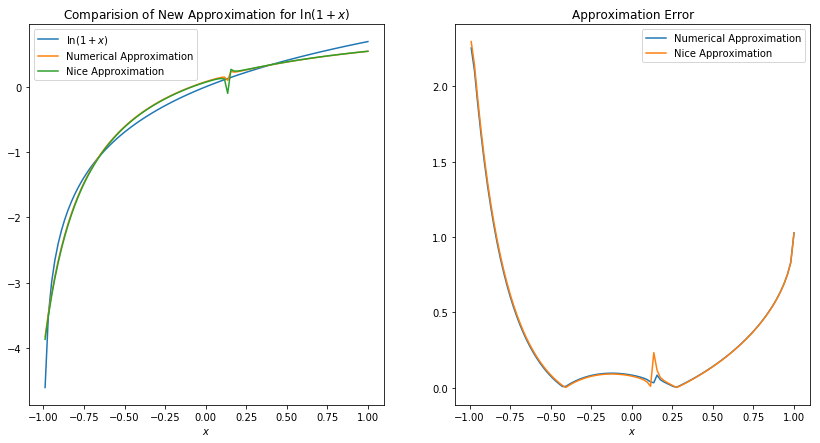Strangely, we have a bump in both the original and nice approximations around $x=0$. This is because the limit of the nice approximation as $x\to0$ is not equal to zero, whereas $\ln\left(1+x\right)=0$ when $x=0$, which is a fairly significant difference.

However, due to the unique way that the function $\ln\left(1+x\right)$ curves all of the coefficients in the approximation need to be non-zero to well capture its behavior even for a small region about $x=0$. In fact, for $\pm\frac{1}{2}$, disregarding the blip around $x=0$ the nice approximation is not too bad, but is certainly not the good fit I was hoping for.

### Conclusion

For many functions, it is possible to find a reasonably good approximation of the form

Furthermore, approximations of this form frequently characterize the behavior of functions with the same accuracy as a high order Taylor series, without the complexity of a higher order Taylor series. I’ve made a table below to summarize my results, but I’ve excluded the approximation for $\ln\left(1+x\right)$ since that was not a very good approximation.

Function Approximation Interval
$\sin\left(\theta\right)$ $\frac{21\theta}{5\theta^2+20}$ just shy of $\left(-\frac{\pi}{2},\frac{\pi}{2}\right)$
$\cos\left(\theta\right)$ $-\frac{24\theta^2-59}{6\theta^2+59}$ $\left(-\frac{\pi}{2},\frac{\pi}{2}\right)$
$\tan\left(\theta\right)$ $\frac{3\theta}{3-\theta^2}$ $\left(-\frac{3\pi}{4},\frac{3\pi}{4}\right)$
$\sin^{-1}\left(x\right)$ $\frac{27x}{29-10x^2}$ just shy of $\left(-1, 1\right)$

It is worth mentioning again that these approximations are designed for high precision use by hand, these methods are not meant to serve as a quick and dirty replacement for the extremely high precision methods used by computers.

A final observation is that for many of the functions, the error of the approximations, both the original and nice ones, seem to oscillate about some center point and then diverge. This is especially apparent in the approximation error graph for $\sin^{-1}\left(x\right)$, where the graph is almost perfectly symmetric about $x=0$. This behavior can also be seen in the approximation error graphs for $\sin\left(\theta\right)$ and $\cos\left(\theta\right)$. This leads me to conjecture that the error in an approximation of the form above for a periodic function will also be periodic about some point $x_0$ so long as the interval the approximation is fit for is of the form $\left(x_0-\delta x, x_0+\delta x\right)$. Unfortunately, I’m not really sure how I would go about proving or disproving such a conjecture at this time.

In conclusion, this exercise actually produced some potentially very helpful approximations and was good practice with scipy’s curve_fit function and numerical error analysis.

A jupyter notebook version of this blog post can be found here.

Tweet me @https://twitter.com/TimTimWillard if you like this post.